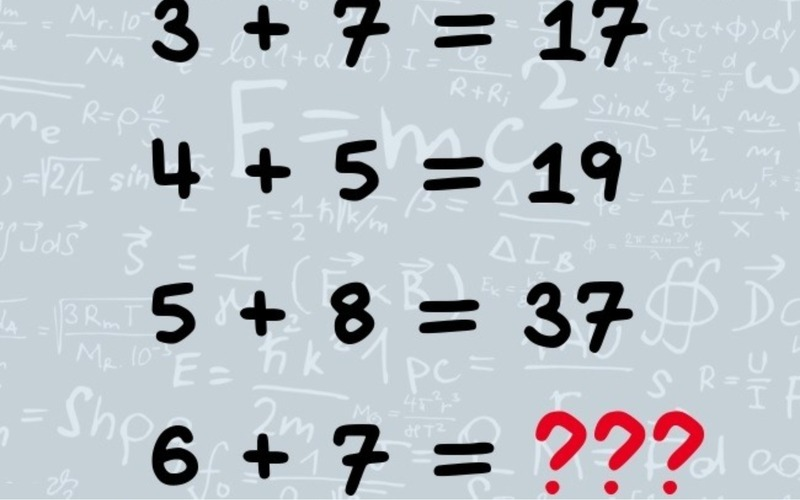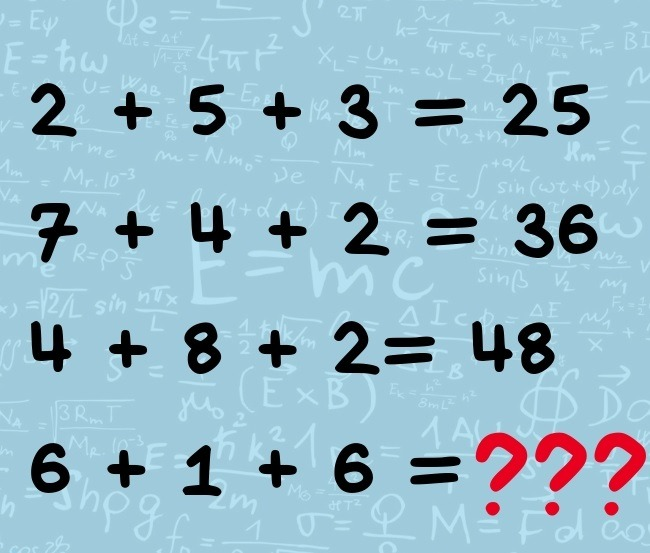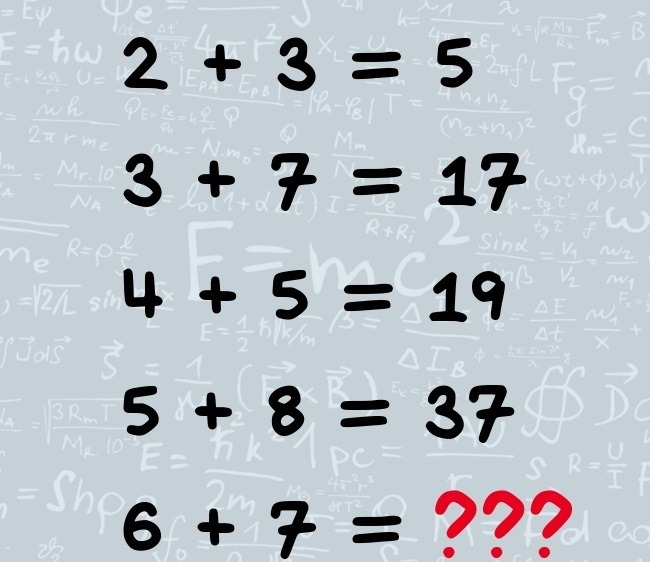# Do you like tasks based on logic? If yes, we have got something to offer you!Can you solve these three easy logical mathematic tasks?

At the end of these three tasks you can find the correct answers with their explanation.

If you are ready, let’s get started!

Test number 1 (the level-easy)

What numeral should be here?Test number 2 (the level-average)

What numeral should be here?Test number 3 (the level- difficult)

What numeral should be here?Test number 1

13  16
25  210
66  612
82  84

The first numeral remains the same, the second one is multiplied by two.

Test number 2

(6+6)*1=12

Test number 3

2+3=1*3-(3-2)=5

3+7=3*7-(7-3)=17

4+5=4*5-(5-4)=19

5+8=5*8-(8-5)=97

6+7=6*7-(7-6)=41